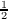#### Vol. 41, No. 2, 1972

 Download this articleFor screen For printingRecent Issues Vol. 325: 1  2 Vol. 324: 1  2 Vol. 323: 1  2 Vol. 322: 1  2 Vol. 321: 1  2 Vol. 320: 1  2 Vol. 319: 1  2 Vol. 318: 1  2Online Archive Volume: Issue:The Journal Subscriptions Editorial Board Officers Contacts Submission Guidelines Submission Form Policies for Authors ISSN: 1945-5844 (e-only) ISSN: 0030-8730 (print) Special Issues Author Index To Appear Other MSP Journals
Stochastic integrals in abstract Wiener space

### Hui-Hsiung Kuo

Vol. 41 (1972), No. 2, 469–483
##### Abstract

Let W(t,ω) be the Wiener process on an abstract Wiener space (i,H,B) corresponding to the canonical normal distributions on H. Stochastic integrals 0tξ(s,ω)dW(s,ω) and 0t(ζ(s,ω), dW(s,ω)) are defined for non-anticipating transformations ξ with values in (B,B) such that (ξ(i,ω) I)(B) B and ζ with values in H. Suppose X(t,ω) = x0+ 0tξ(s,ω)dW(s,ω) + 0tσ(s,ω)ds, where σ is a non-anticipating transformation with values in H. Let f(t,x) be a continuous function on R × B, continuously twice differentiable in the H-directions with D2f(t,x) ∈ℬ1(H,H) for the x variable and once differentiable for the t variable. Then f(t,X(t,ω)) = f(0,x0) + 0tξ(s,ω)Df(s,X(s,ω))dW(s,ω) + 0t{∂f∕∂s(s,X(s,ω)) + Df(s,X(s,ω))(s,ω)+trace[ξ(s,ω)D2f(s,X(s,ω))ξ(s,ω)]}ds, where ,is the inner product of H. Under certain assumptions on A and σ it is shown that the stochastic integral equation X(t,ω) = x0 + 0tA(X(s,ω))dW(s,ω) + 0tσ(X(s,ω))ds has a unique solution. This solution is a homogeneous strong Markov process.

Primary: 28A40
Secondary: 60H05
##### Milestones